# Generics in C#

## Generics in C# with Examples

In this article, I am going to discuss how to implement Generics in C# with Examples. Please read our previous article where we discussed the Generic Collection in C#. In C#, Generic means not specific to a particular data type, that type will be decided by the compiler at the time of compilation. As part of this article, we are going to discuss the following pointers.

1. Why do we need Generics in C#?
2. What are Generics in C#?
3. Advantages of Generics in C#.
4. How to implement Generics in C# with Examples?
5. How to use Generics with Class and its Members in C#?
##### Why do we need Generics in C#?

Generic is a concept that allows us to define classes and methods with placeholders. C# Compiler replaces these placeholders with the specified type at compile time. The concept of generics is used to create general-purpose classes and methods.

Let us understand the need for Generics in C# with one example. Let us create a simple program to check whether two integer numbers are equal or not. The following code implementation is very straightforward. Here we created two classes with the name ClsCalculator and ClsMain. Within the ClsCalculator class, we have AreEqual() method which takes two integer values as the input parameter and then it checks whether the two input values are equal or not. If both are equal then it returns true else it will return false. And from the ClsMain class, we are calling the static AreEqual() method and showing the output based on the return value.

```using System;
namespace GenericsDemo
{
public class ClsMain
{
private static void Main()
{
bool IsEqual = ClsCalculator.AreEqual(10, 20);
if (IsEqual)
{
Console.WriteLine("Both are Equal");
}
else
{
Console.WriteLine("Both are Not Equal");
}

}
}

public class ClsCalculator
{
public static bool AreEqual(int value1, int value2)
{
return value1 == value2;
}
}
}```

The above AreEqual() method works as expected as it is and more importantly it will only work with the integer values as this is our initial requirement. Suppose our requirement changes, now we also need to check whether two string values are equal or not.

In the above example, if we try to pass values other than the integer values, then we will get a compile-time error. This is because the AreEqual() method of the ClsCalculator class is tightly bounded with the integer data type and hence it is not possible to invoke the AreEqual method other than the integer data type values. So, when we try to invoke the AreEqual() method by passing string values as shown below we get a compile-time error.

bool Equal = ClsCalculator.AreEqual(“ABC”, “XYZ”);

One of the ways to make the above AreEqual() method to accepts string type values as well as integer type values, we need to make use of the object data type as the parameters. If we make the parameters of the AreEqual() method as the Object data type, then it is going to work with any data type.

Note: The most important point that you need to keep in remember is every .NET data type whether it is a primitive type or reference type, directly or indirectly inherits from the System.Object data type.

##### Modifying the AreEqual() Method to Accept Any Data Type Values:

Let’s modify the AreEqual() method of the ClsCalculator class to use the Object data type, so that it can accept any type of values as shown below.

```using System;
namespace GenericsDemo
{
public class ClsMain
{
private static void Main()
{
// bool IsEqual = ClsCalculator.AreEqual(10, 20);
bool IsEqual = ClsCalculator.AreEqual("ABC", "ABC");
if (IsEqual)
{
Console.WriteLine("Both are Equal");
}
else
{
Console.WriteLine("Both are Not Equal");
}
}
}

public class ClsCalculator
{
//Now this method can accept any data type
public static bool AreEqual(object value1, object value2)
{
return value1 == value2;
}
}
}```

That’s it. Run the application and you will see it is working as expected. Let’s see the problem of the above code implementation.

1. We get poor Performance due to boxing and unboxing. The object type needs to be converted to the value type.
2. Now, the AreEuqal() method is not type-safe. Now it is possible to pass a string value for the first parameter and an integer value for the second parameter.

Another option is we need to overload the AreEqual method which will accept different types of parameters as shown below. As you can see in the below code, now we have created three different methods with the same name but with different types of parameters. This is nothing but method overloading. Now, run the application and you will see everything is working as expected.

```using System;
namespace GenericsDemo
{
public class ClsMain
{
private static void Main()
{
// bool IsEqual = ClsCalculator.AreEqual(10, 20);
// bool IsEqual = ClsCalculator.AreEqual("ABC", "ABC");
bool IsEqual = ClsCalculator.AreEqual(10.5, 20.5);

if (IsEqual)
{
Console.WriteLine("Both are Equal");
}
else
{
Console.WriteLine("Both are Not Equal");
}

}
}

public class ClsCalculator
{
public static bool AreEqual(int value1, int value2)
{
return value1 == value2;
}

public static bool AreEqual(string value1, string value2)
{
return value1 == value2;
}

public static bool AreEqual(double value1, double value2)
{
return value1 == value2;
}
}
}```

The problem with the above code implementation is that we are repeating the same logic in each and every method. However, if tomorrow we need to compare two float or two long values then again, we need to create two more methods. I do not want to repeat the logic again and again i.e. I need only one method with the logic and if two tomorrow, we need to compare two floats or two long values, then my method should do this. What is the solution?

##### How to Solve the Above Problems in C#?

We can solve the above problems with Generics in C#. With generics, we will make the AreEqual() method to works with different types of data. Let us first modify the code implementation to use the generics and then we will discuss how it works.

```using System;
namespace GenericsDemo
{
public class ClsMain
{
private static void Main()
{
//bool IsEqual = ClsCalculator.AreEqual<int>(10, 20);
//bool IsEqual = ClsCalculator.AreEqual<string>("ABC", "ABC");
bool IsEqual = ClsCalculator.AreEqual<double>(10.5, 20.5);
if (IsEqual)
{
Console.WriteLine("Both are Equal");
}
else
{
Console.WriteLine("Both are Not Equal");
}
}
}

public class ClsCalculator
{
//public static bool AreEqual(int value1, int value2)
//{
//    return value1 == value2;
//}

public static bool AreEqual<T>(T value1, T value2)
{
return value1.Equals(value2);
}
}
}
```

Here in the above example, in order to make the AreEqual() method generic (generic means the same method will work with the different data types), we specified the type parameter T using the angular brackets <T>. Then we use that type as the data type for the method parameters as shown in the below image.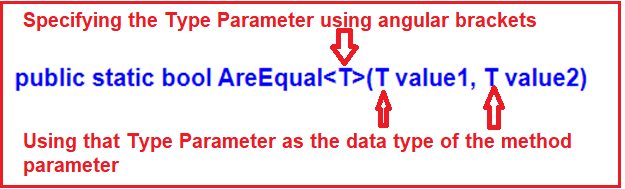At this point, if you want to invoke the above AreEqual() method, then you need to specify the data type on which the method should operate. For example, if you want to work with integer values, then you need to invoke the AreEqual() method by specifying int as the data type as shown in the below image using angular brackets.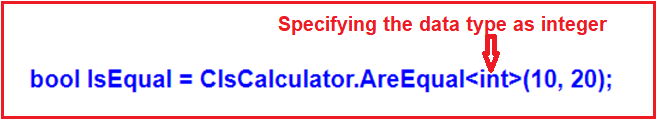The above AreEqual() generic method is working as follows: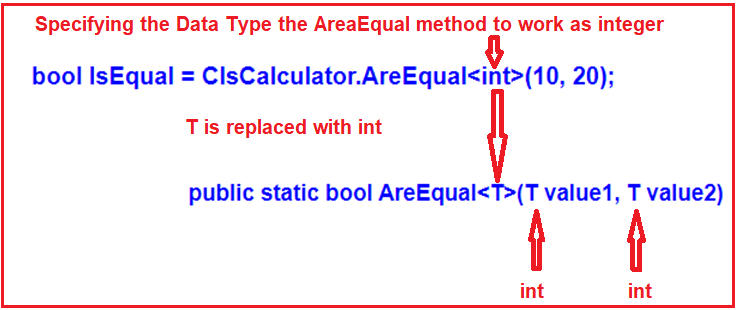If you want to work with the string values, then you need to call the AreEqual() method as shown below.
bool IsEqual= ClsCalculator.AreEqual<string>(“ABC”, “ABC”);

Now, I hope you understand the need and importance of Generics in C#.

##### What are Generics in C#?

As we already discussed, the Generics are introduced as part of C# 2.0. The Generics allow us to define classes and methods which are decoupled from the data type. In other words, we can say that the Generics allow us to create classes using angular brackets specifying the data type of its members. At compilation time, these angular brackets are going to be replaced with some specific data types. In C#, the Generics can be applied to the following:

1. Interface
2. Abstract class
3. Class
4. Method
5. Static method
6. Property
7. Event
8. Delegates
##### Advantages of Generics in C#
1. It Increases the Reusability of the Code: For example, you can create a generic method to add two numbers. This method can be used to add two integers as well as two float numbers without any modification to the code.
2. Generics are Type-Safe: Generics are type safety, especially in the case of collections. When using generics, we need to define the type of objects to be passed to a collection. This helps the compiler to ensure that only those object types that are defined in the definition can be passed to the collection. We will get the compile-time error if we try to use a different type of data rather than the one, we specified in the definition.
3. Generic Provides Better Performance: It gives better performance compared to non-Generics because they reduce the need for boxing, unboxing, and typecasting of variables or objects.
##### How to use Generics with Class and its Members in C#?

Let us create a generic class with a generic constructor, generic member variable, generic property, and a generic method. Please create a class file with the name MyGenericClass.cs and then copy and paste the following code into it. The following Example code is self-explained, so please go through the comment lines.

```using System;
namespace GenericsDemo
{
//MyGenericClass is a Generic Class
//Here T specifies the Data Types of the class Members
class MyGenericClass<T>
{
//Generic variable
//The data type is generic i.e. T
private T GenericMemberVariable;

//Generic Constructor
//Constructor accepts one parameter of Generic type i.e. T
public MyGenericClass(T value)
{
GenericMemberVariable = value;
}

//Generic Method
//Method accepts one Generic type Parameter i.e. T
//Method return type also Generic i.e. T
public T GenericMethod(T GenericParameter)
{
Console.WriteLine(\$"Parameter type: { typeof(T).ToString()}, Value: {GenericParameter}");
Console.WriteLine(\$"Return type: {typeof(T).ToString()}, Value: {GenericMemberVariable}");
return GenericMemberVariable;
}

//Generic Property
//The data type is generic i.e. T
public T GenericProperty { get; set; }
}
}
```

In the above example, we created the class MyGenericClass with parameter <T>. The angular brackets (<T>) indicate that MyGenericClass is a generic class and the type for this <T> is going to be defined later. Later we need to specify the T while creating an instance of the MyGenericClass class. You can specify the T data type as primitive types such as int, float, long, etc., or reference types such as String, Object, Customer, Employee, etc.

So, while creating the instance of this MyGenericClass class, we need to specify the type and the compiler will assign that type to T. In the following example, we use int as the data type. Once we create an instance of the MyGenericClass, then we are invoking the GenericMethod method. As we have specified the T as int while creating the instance, we do not need to specify the same while invoking the class members.

```using System;
namespace GenericsDemo
{
class Program
{
static void Main()
{
MyGenericClass<int> integerGenericClass = new MyGenericClass<int>(10);
int val = integerGenericClass.GenericMethod(200);
Console.WriteLine(val);
}
}
}
```

Run the application and it will give you the following output.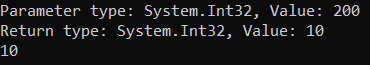The following diagram shows how the T will be replaced with the int data type by the compiler.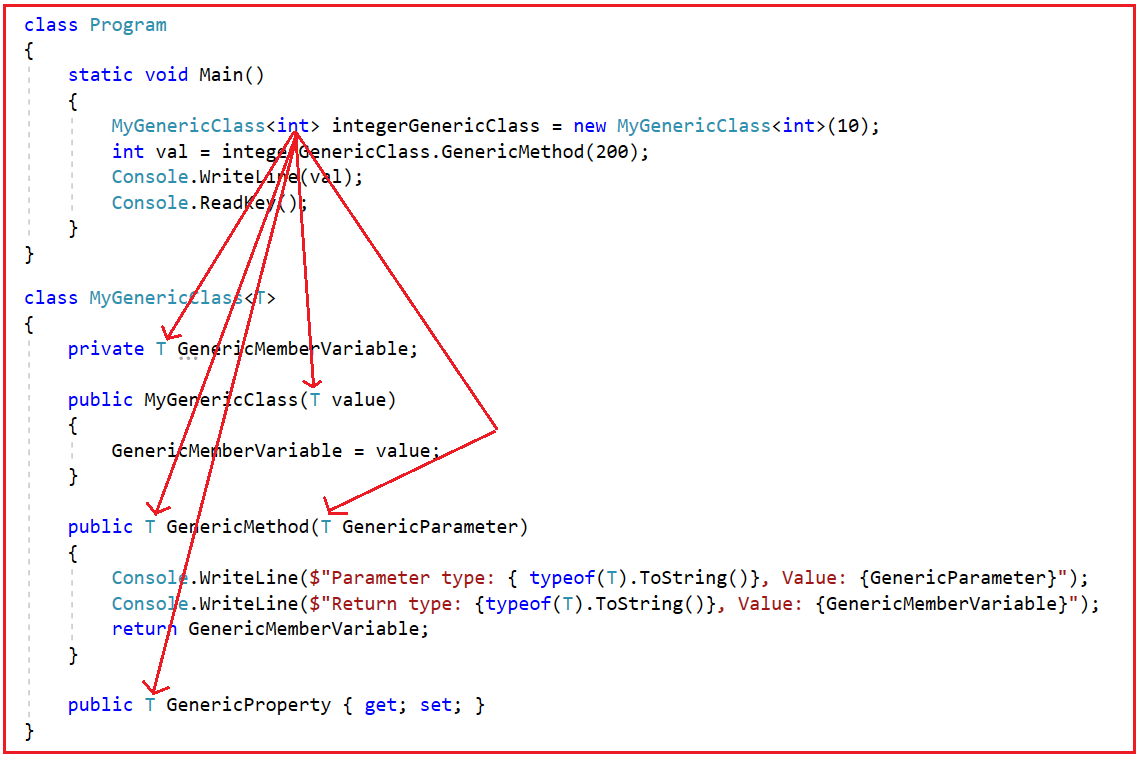The compiler will compile the above class for the class instantiation with int data type as shown in the below image.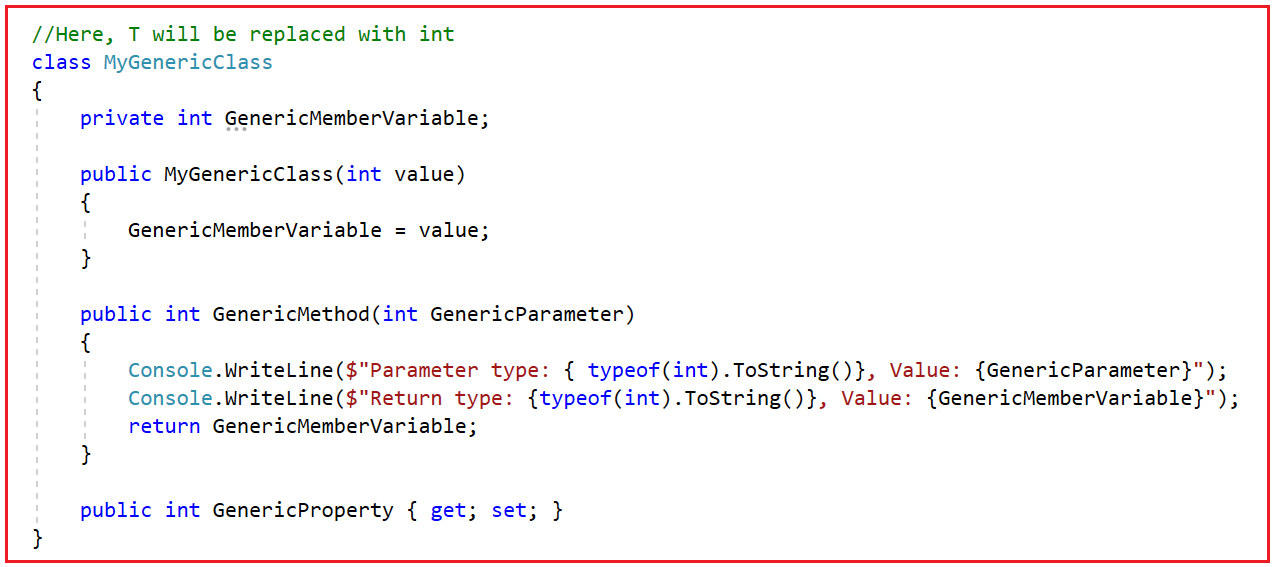At the time of instantiation, you can use any type as per your requirement. If you want to use a string type, then you need to instantiate the class as shown below

```using System;
namespace GenericsDemo
{
class Program
{
static void Main()
{
MyGenericClass<string> stringGenericClass = new MyGenericClass<string>("Hello Generic World");
stringGenericClass.GenericProperty = "This is a generic property example.";
string result = stringGenericClass.GenericMethod("Generic Parameter");
Console.WriteLine(result);
}
}
}
```
###### Output: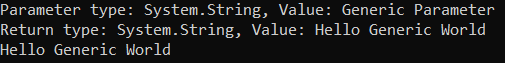Now, I hope you understand the concept of Generics in C#. The generics are extremely used by the collection classes which belong to System.Collections.Generic namespace. Now, let us see a few examples to understand how to use Generics with Classes, Fields, and Methods in C#.

##### Generic Class Example in C#

The following example shows how to create a generic class using type parameter (T) with angle (<>) brackets in the C# language. In the below example, we are creating the class with <T> type and then we have created one variable and method using the T parameter. Then while creating the instance we specified the T type as a string. So, at the time of compilation, wherever you used T inside the GenericClass, that will be replaced with the string data type.

```using System;
namespace GenericsDemo
{
public class GenericClass<T>
{
public T Message;
public void GenericMethod(T Name, T Location)
{
Console.WriteLine(\$"Message: {Message}");
Console.WriteLine(\$"Name: {Name}");
Console.WriteLine(\$"Location: {Location}");
}
}
class Program
{
static void Main(string[] args)
{
Console.WriteLine("Generics Class Example in C#");
//Instantiate GenericClass, string is the type argument
GenericClass<string> myGenericClass = new GenericClass<string>
{
Message = "Welcome to DotNetTutorials"
};
myGenericClass.GenericMethod("Pranata Rout", "Bhubaneswar");
}
}
}
```

Now, when you execute the above code, you will get the following output.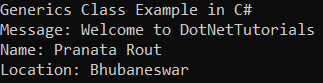##### Generic Method Example in C#:

It is also possible in C# to define a method as generic i.e. we can define the <T> while creating the method. In that case, we can call the generic method passing any type of argument. For a better understanding, please have a look at the below example which shows how to create and call a Generic Method in C#. Please note here we are not making the class Generic rather we are only making the method generic. In the below example, we are making the Generic Method work with two generic parameters. So, while calling the method we need to specify the type for both T1 and T2.

```using System;
namespace GenericsDemo
{
public class SomeClass
{
//Making the Method as Generic by using the <T1, T2>
//Then using T1 and T2 as parameters of the method
public void GenericMethod<T1, T2>(T1 Param1, T2 Param2)
{
Console.WriteLine(\$"Parameter T1 type: {typeof(T1)}: Parameter T2 type: {typeof(T2)}");
Console.WriteLine(\$"Parameter 1: {Param1} : Parameter 2: {Param2}");
}
}

class Program
{
static void Main(string[] args)
{
Console.WriteLine("Generic Method Example in C#");
SomeClass s = new SomeClass();
//While calling the method we need to specify the type for both T1 and T2
s.GenericMethod<int, int>(10, 20);
s.GenericMethod<double, string>(10.5, "Hello");
s.GenericMethod<string, float>("Anurag", 20.5f);
}
}
}
```

Now, when you execute the above code, you will get the following output.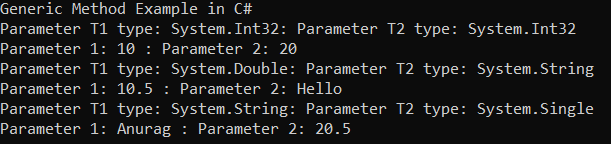##### Generic Field Example in C#:

A generic class can include generic fields of different generic types. For a better understanding, please have a look at the below example which shows how to create and use a Generic Field in C#. In the below example, we are defining two generic types i.e. T1 and T2. While creating the instance, we need to specify the types for both T1 and T2.

```using System;
namespace GenericsDemo
{
public class GenericClass<T1, T2>
{
public GenericClass(T1 Parameter1, T2 Parameter2)
{
Param1 = Parameter1;
Param2 = Parameter2;
}
public T1 Param1 { get; }
public T2 Param2 { get; }
}

class Program
{
static void Main()
{
var obj1 = new GenericClass<int, string>(100, "One Hundred");
Console.WriteLine(\$"{obj1.Param1} : {obj1.Param2}");

var obj2 = new GenericClass<string, string>("Dot Net Tutorails", "Welcome to C#.NET");
Console.WriteLine(\$"{obj2.Param1} : {obj2.Param2}");

var obj3 = new GenericClass<int, int>(100, 200);
Console.WriteLine(\$"{obj3.Param1} : {obj3.Param2}");

}
}
}
```

Now, when you execute the above code, you will get the following output.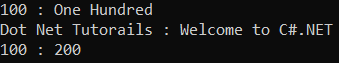### 7 thoughts on “Generics in C#”

1.this is awesome and very clear explanation for beginner, thank you

2.3.Your all topics are superb ..thanks

4.Your explanation is good .One observation ,method overloading can be achieved within same classes and not in different classes.

5.Md.Selim Shaikh

I am Surprised. Thank u so much for this knowledgeable article.

6.Many thanks. First article I’ve found that explains why Generics is so useful and how it works in detail.